# Sample Data: Gorilla Nesting Sites

Locations of gorilla nesting sites annotated with group (major/minor), season (dry/rainy), and date marks

## Details

Locations of gorilla nesting sites in a polygonal observation region bounded by the Rectangle[{580458., 674173.}, {585934., 678739.}] meters, annotated with group (major/minor), season (dry/rainy), and date marks.

## Examples

### Basic Examples (1)

 In:=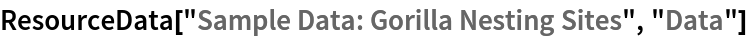Out=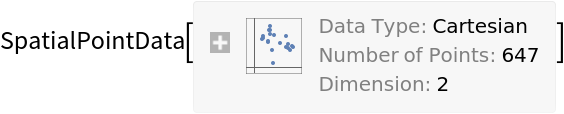Summary of the spatial point data:

 In:=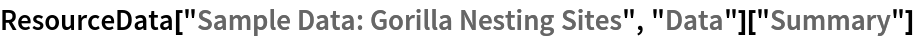Out=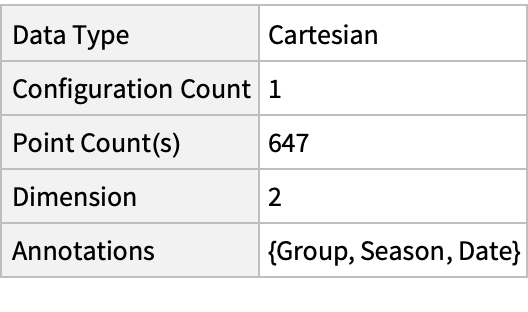### Visualizations (2)

Plot the spatial point data:

 In:=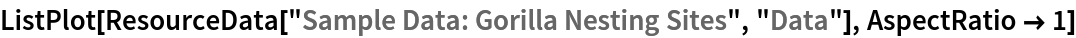Out=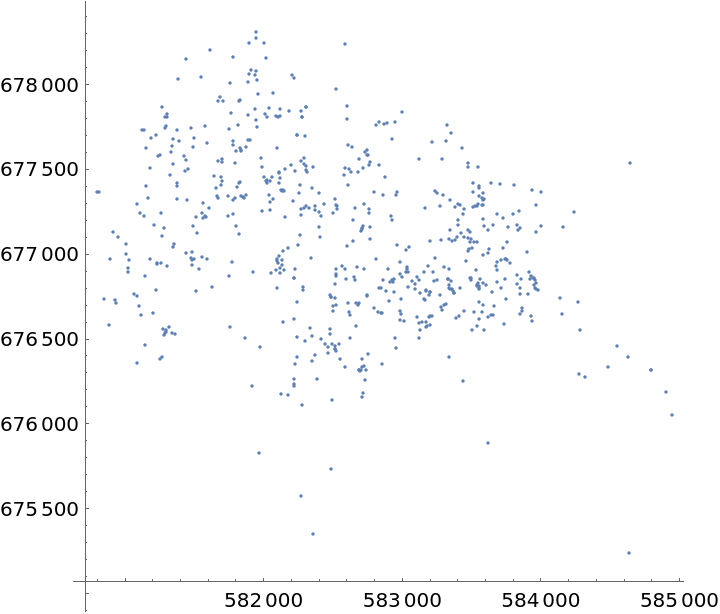Visualize smooth point density:

 In:=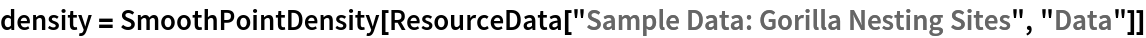Out=In:=Out=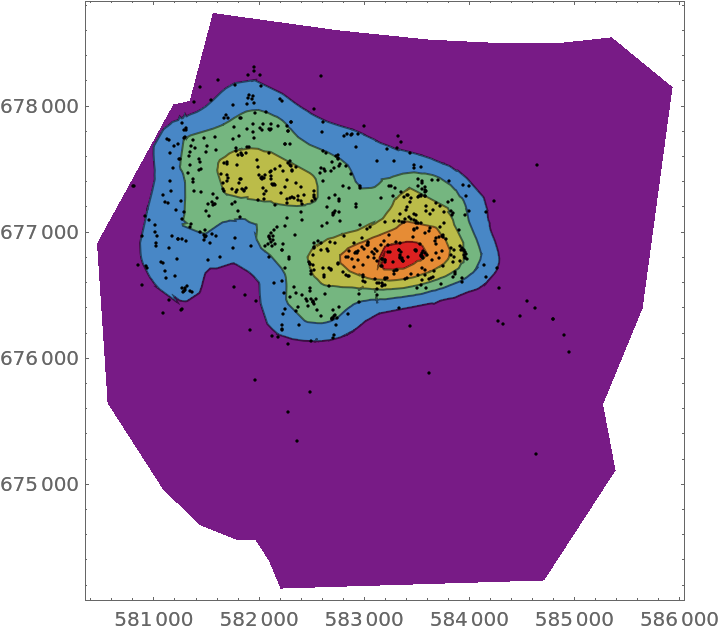### Analysis (3)

Compute probability of finding a point within given radius of an existing point - NearestNeighborG is the CDF of the nearest neighbor distribution:

 In:=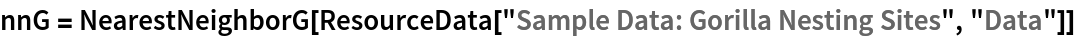Out=In:=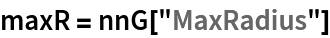Out=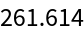In:=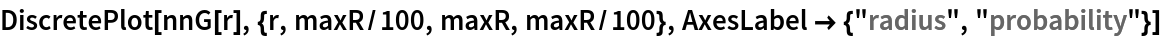Out=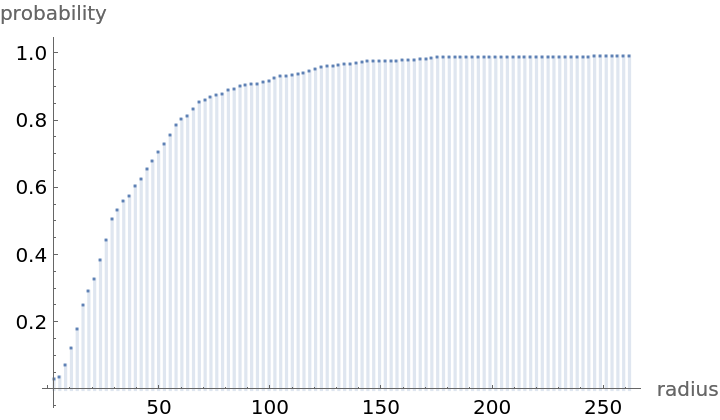Mean distance between a typical point and its nearest neighbor (for positive support distribution can be approximated via a Riemann sum of 1-CDF):

 In:=In:=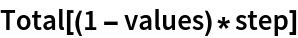Out=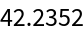Test for complete spatial randomness:

 In:=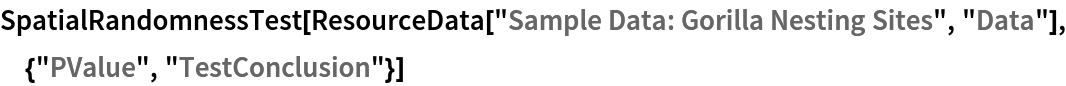Out=Gosia Konwerska, "Sample Data: Gorilla Nesting Sites" from the Wolfram Data Repository (2021)